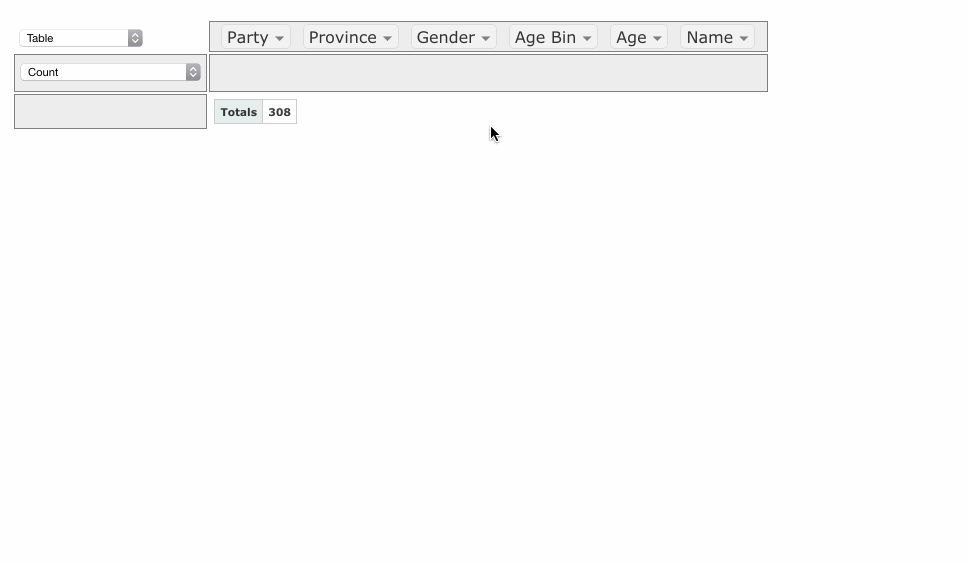# Interactive Pivot Tables in Jupyter Notebook

Original article was published by Satyam Kumar on Artificial Intelligence on Medium# Interactive Pivot Tables in Jupyter Notebook

## Create interactive pivot table using PivotTable.js

Python has achieved popularity in a short span of time, still, it falls short to interact with data in some cases, that is where excel takes a lead. For the development of a model, the initial steps are data understanding and Exploratory data analysis (EDA).

For EDA you need the ability of flexibility to play around with data. Python falls short in terms of interacting with the data in case of creating pivot tables. For creating pivot tables, Excel takes a lead, as it comes up with interactive and dynamic development of tables.

Creating a pivot table in pandas is a bit combustion process. The structure of the pivot table needs to be decided in advance and accordingly, the script needs to be developed. To make some manipulation in the pivot table requires to change the code again and run the script. This is a combustion process to generate insights as to proceed with model building.

`pd.pivot_table(df,                values='Survived',               index=['Pclass'],               columns=['Sex'],               aggfunc='sum'              )`
`pd.pivot_table(df,                values='Survived',               index=['Pclass','Embarked'],               columns=['Sex'],               aggfunc='sum'              )`

Pivot tables are used to get insights about the data during EDA, to generate pivot tables in pandas, code needs to be manipulated for each table, which makes it a bit of combustion.

Here, PivotTable.js comes in the picture, and it helps to create interactive pivot tables.

# PivotTable.js:

PivotTable.js is an open-source Javascript Pivot Table (aka Pivot Grid, Pivot Chart, Cross-Tab) implementation with drag’n’ drop functionality.

## Installation:

`pip install pivottablejs`

## Usage:

Now trying to generate the same pivot tables as developed using pandas earlier in this article.

## Features:

• PivotTable.js provides the privilege of choosing the aggregate functions from the drop-down list.
• Different representation of pivot tables, and heatmaps.
• Several types of plots including bar plot, stacked bar plot, line chart, area chart, scatter chart, treemap, etc.